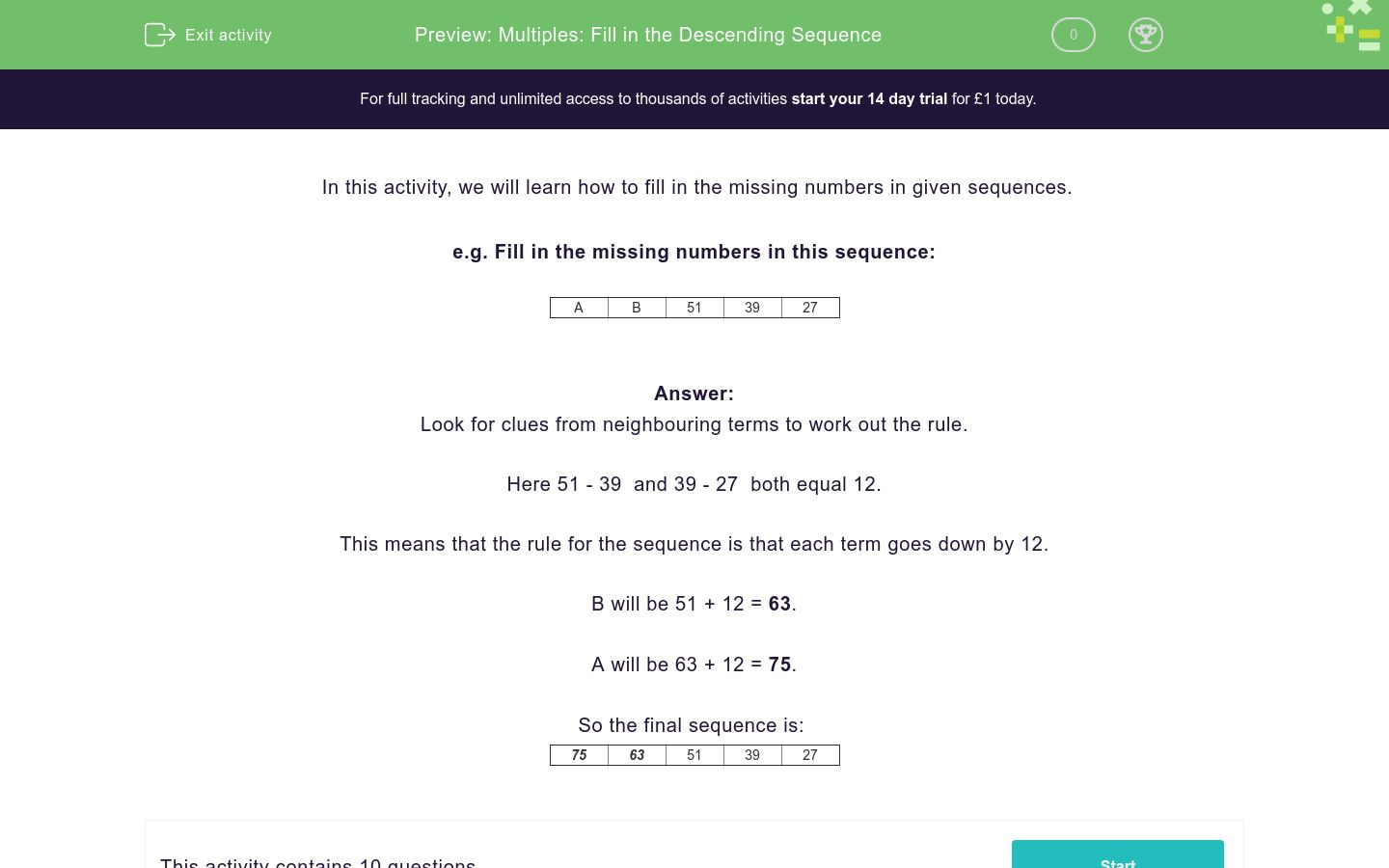# Multiples: Fill in the Descending Sequence

In this worksheet, students use work out a rule to help them fill in the missing numbers of a descending sequence.Key stage:  KS 2

Curriculum topic:   Number: Multiplication and Division

Curriculum subtopic:   Identify Multiples and Factors

Difficulty level:### QUESTION 1 of 10

In this activity, we will learn how to fill in the missing numbers in given sequences.

e.g. Fill in the missing numbers in this sequence:

 A B 51 39 27

Look for clues from neighbouring terms to work out the rule.

Here 51 - 39  and 39 - 27  both equal 12.

This means that the rule for the sequence is that each term goes down by 12.

B will be 51 + 12 = 63.

A will be 63 + 12 = 75.

So the final sequence is:

 75 63 51 39 27

Fill in the missing numbers in this sequence.

 A 59 45 31 B

Fill in the missing numbers in this sequence.

 79 71 A B 47

Fill in the missing numbers in this sequence.

 A 68 B 44 32

Fill in the missing numbers in this sequence.

 84 A B 51 40

Fill in the missing numbers in this sequence.

 60 47 A 21 B

Fill in the missing numbers in this sequence.

 84 70 A 42 B

Fill in the missing numbers in this sequence.

 79 69 59 A B

Fill in the missing numbers in this sequence.

 A 65 B 45 35

Fill in the missing numbers in this sequence.

 82 71 A B 38

Fill in the missing numbers in this sequence.

 A B 36 21 C

## Column B

A
51
B
66
C
6
• Question 1

Fill in the missing numbers in this sequence.

 A 59 45 31 B
73
17
EDDIE SAYS
A. 59 + 14 = 73 B. 31 - 14 = 17
• Question 2

Fill in the missing numbers in this sequence.

 79 71 A B 47
63
55
EDDIE SAYS
A. 71 - 8 = 63 B. 63 - 8 = 55
• Question 3

Fill in the missing numbers in this sequence.

 A 68 B 44 32
80
56
EDDIE SAYS
A. 68 + 12 = 80 B. 44 + 12 = 56
• Question 4

Fill in the missing numbers in this sequence.

 84 A B 51 40
73
62
EDDIE SAYS
A. 84 - 11 = 73 B. 73 - 11 = 62
• Question 5

Fill in the missing numbers in this sequence.

 60 47 A 21 B
34
8
EDDIE SAYS
A. 47 - 13 = 34 B. 21 - 13 = 8
• Question 6

Fill in the missing numbers in this sequence.

 84 70 A 42 B
56
28
EDDIE SAYS
A. 70 - 14 = 56 B. 42 - 14 = 28
• Question 7

Fill in the missing numbers in this sequence.

 79 69 59 A B
49
39
EDDIE SAYS
A. 59 - 10 = 49 B. 49 - 10 = 39
• Question 8

Fill in the missing numbers in this sequence.

 A 65 B 45 35
75
55
EDDIE SAYS
A. 65 + 10 = 75 B. 45 + 10 = 55
• Question 9

Fill in the missing numbers in this sequence.

 82 71 A B 38
60
49
EDDIE SAYS
A. 71 - 11 = 60 B. 60 - 11 = 49
• Question 10

Fill in the missing numbers in this sequence.

 A B 36 21 C

## Column B

A
66
B
51
C
6
EDDIE SAYS
A. 51 + 15 = 66 B. 36 + 15 = 51 C. 21 - 15 = 6
---- OR ----

Sign up for a £1 trial so you can track and measure your child's progress on this activity.

### What is EdPlace?

We're your National Curriculum aligned online education content provider helping each child succeed in English, maths and science from year 1 to GCSE. With an EdPlace account you’ll be able to track and measure progress, helping each child achieve their best. We build confidence and attainment by personalising each child’s learning at a level that suits them.

Get started